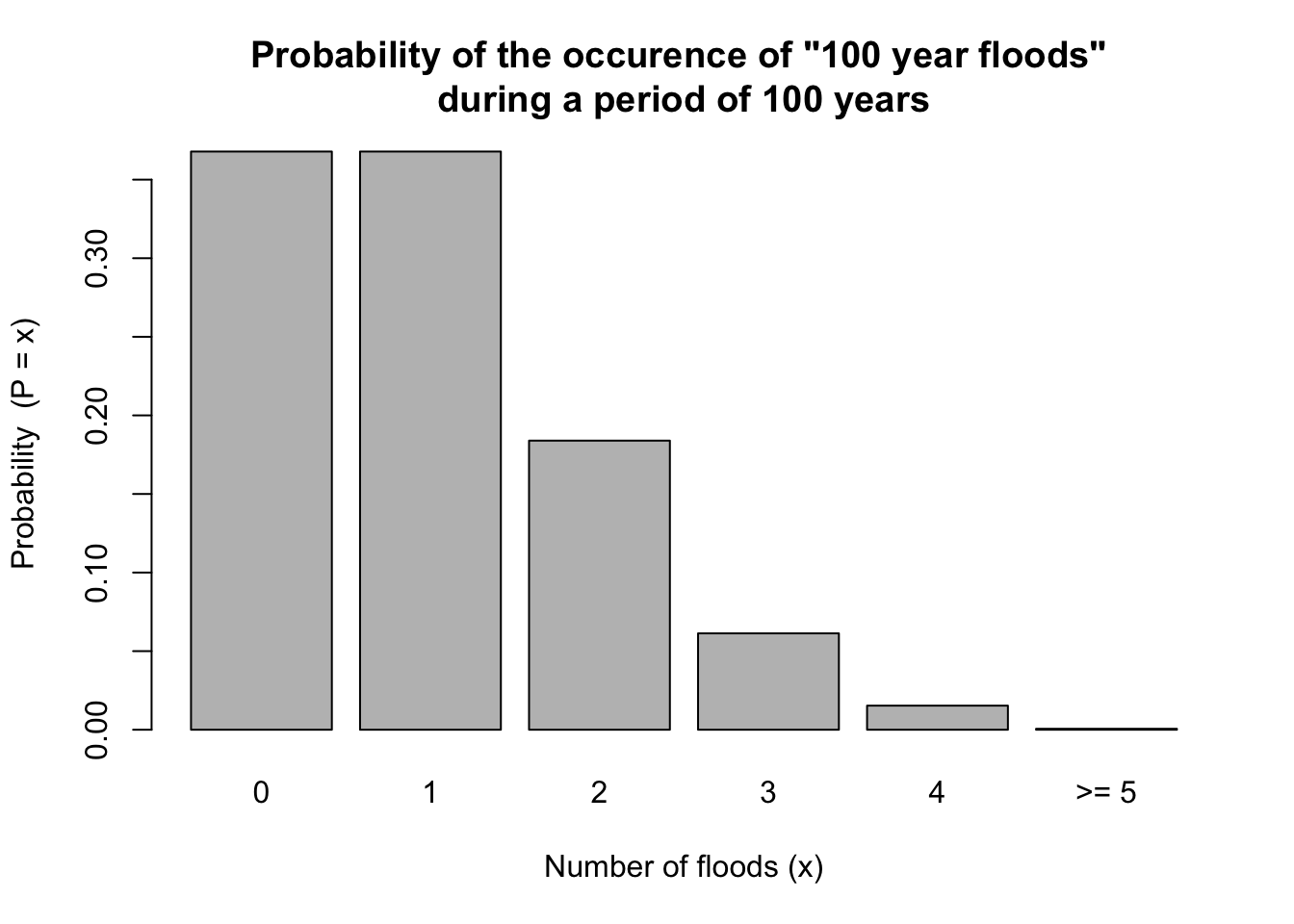Let us apply the Poisson Distribution if form of an example. We focus on the one-hundred-year flood, a concept that is widely used in river engineering to design flood control measures.

Let us recall the mathematical notation of a poisson random variable:

$P(X = x) = e^{-\lambda}\frac{\lambda x}{x!}, \qquad x = 0, 1, 2, . . . ,$

where $$\lambda$$ is a positive real number, which represents the average number of events occurring during a fixed time interval, and $$e \approx 2.7182818$$.

The 100-year flood is a shorthand term for a flood with an annual exceedance probability of 1% and an average recurrence interval of 100 years. However, the term can be confusing for people because, not unreasonably, as they imagine the term describes floods, which occur once every 100-years. However, this is not true. A flood with an annual exceedance probability of 1% indicates that a with a probability of 0.01 a flood of a magnitude corresponding to a 100 year flood occurs in any given year.

Putting this in the context of a Poisson distribution the expected value, $$E(x) = \lambda$$, of such a flood during the fixed interval of 100 years is set to $$\lambda= 100\times 0.01 = 1$$. Consequently, the Poisson random variable $$X$$ is the number of occurrences, which of course may take several values depending our question. We may be interested in the probability that such an flood event will not occur during the 100-year interval $$P(x=0)$$, or we want to know the probability the such an flood event will occur exactly once during the 100-year interval, thus $$P(x=1)$$, or we want to know the probability that two or more of such flood events occur during the 100-year interval, thus $$P(x \ge 2)$$. Plugging these values in the equation from above yields

$$\lambda = 1, x = 0,1,2,..,n$$

$P(X = 0) = e^{-1}\frac{1 \times 0}{0!}, \qquad \text{for } x = 0$ $P(X = 1) = e^{-1}\frac{1 \times 1}{1!}, \qquad \text{for } x = 1$ $P(X \ge 2) = \sum_{i=2}^n e^{-1}\frac{1 \times x_i}{x_i!}, \qquad \text{for } x_i = 2,3,...,n$

We turn to R to do the calculations. We will make us of the dpois() and the ppois() function.

lambda = 1 # set expected value
x = c(0,1) # set  Poisson random variables  of interest

dpois(x = x, lambda = lambda)
##  0.3678794
dpois(x = x, lambda = lambda)
##  0.3678794
ppois(q = x, lambda = lambda, lower.tail = FALSE) # recall: lower.tail = TRUE, probabilities are P[X <= x], otherwise, P[X > x].
##  0.2642411

The results indicate that the probability that no flood $$P(X = 0)$$ of a magnitude corresponding to a 100 year flood will occur during a period of 100 years is 0.37, which is interestingly as likely as the occurrence of exactly one flood $$P(X = 1)$$. The probability that two or more $$(P(X \ge 2))$$ of such flood events occur during the 100-year interval is 0.26, and thus lower. However, please note that the probability that two or more $$(P(X \ge 2))$$ of such flood events occur during the 100-year interval is approximately 26%!

For a sanity check we sum up the probabilities $$P(x=0)$$, $$P(x=1)$$, and $$P(x \ge 2)$$, which should yield 1,

dpois(x = x, lambda = lambda) +
dpois(x = x, lambda = lambda) +
ppois(q = x, lambda = lambda, lower.tail = FALSE)
##  1

For a better intuition we plot the probabilities of the Poisson random variable $$x = 0,1,2,3,4,\ge 5$$.

lambda = 1 # set expected value
out = NULL
out = dpois(x=0, lambda = lambda)
out = dpois(x=1, lambda = lambda)
out = dpois(x=2, lambda = lambda)
out = dpois(x=3, lambda = lambda)
out = dpois(x=4, lambda = lambda)
out = ppois(q=5, lambda = lambda, lower.tail = FALSE)

#plot
barplot(out,
ylab = paste('Probability ', expression((P=x))),
xlab = 'Number of floods (x)',
names = paste(c("0","1","2","3","4",">= 5")),
main = 'Probability of the occurence of "100 year floods" \nduring a period of 100 years'
)